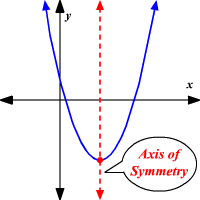# What is the axis of symmetry and vertex for the graph y = 2x^2 - 4x - 6?

Dec 23, 2017

Axis of symmetry: $x = 1$
Vertex: $\left(1 , - 8\right)$

#### Explanation:

$y = 2 {x}^{2} - 4 x - 6$

This equation is a quadratic equation, meaning that it will form a parabola on the graph.

Our equation is in standard quadratic form, or $y = a {x}^{2} + b x + c$.

The axis of symmetry is the imaginary line that runs through the graph where you can reflect it, or have both halves of the graph match.

Here is an example of an axis of symmetry:https://www.varsitytutors.com

The equation to find the axis of symmetry is $x = - \frac{b}{2 a}$.
In our equation, $a = 2$, $b = - 4$, and $c = - 6$.

So let's plug in our $a$ and $b$ values into the equation:
$x = - \frac{- 4}{2 \left(2\right)}$
$x = \frac{4}{4}$
$x = 1$
So our axis of symmetry is $x = 1$.

Now, we need to find the vertex. The vertex is the minimum or maximum point on a quadratic function, and its x-coordinate is the same as the axis of symmetry.

Here's are a couple examples of vertices:http://tutorial.math.lamar.edu/

Since we already found our axis of symmetry, $x = 1$, that is our x-coordinate of the vertex.

To find the y-coordinate of the vertex, we plug that value back into the original quadratic equation for $x$:
$y = 2 {x}^{2} - 4 x - 6$
$y = 2 {\left(1\right)}^{2} - 4 \left(1\right) - 6$
$y = 2 \left(1\right) - 4 - 6$
$y = 2 - 4 - 6$
$y = - 8$

Therefore, our vertex is at $\left(1 , - 8\right)$.

As an extra, here's the graph of this quadratic equation:As you can see, the vertex of the graph is at $\left(1 , - 8\right)$, as we solved.

Hope this helps!